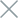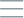• Home
• Tags
• Series
•# Getting Started with Modules and Packages in Python

Modules and packages are two important concepts in Python for organizing and managing source code. Here is a description of modules and packages and how to use them:

## Module

• In Python, a module is a collection of definitions, functions, variables, and statements that are written to be used.
• Each Python file can be considered a module and contains code related to a specific functionality.
• You can use built-in Python modules or create your own modules to use in your code.

Example: Create a file named `math_operations.py` containing some math functions:

``````# math_operations.py
return a + b

def subtract(a, b):
return a - b

def multiply(a, b):
return a * b

def divide(a, b):
return a / b
``````

Then, you can use these functions in another program by importing the `math_operations` module:

``````# main.py
import math_operations

print(result)   # Output: 15
``````

## Package

• A package is a way to organize and group related modules together.
• It is a directory that contains Python files (modules) and an empty `__init__.py` file to indicate that the directory is a package.
• Packages help organize your code into logical scopes and structured directories.

Example: Create a package named `my_package`, containing two modules `module1.py` and `module2.py`:

``````my_package/
__init__.py
module1.py
module2.py
``````

In `module1.py`, we have the following code:

``````# module1.py
def greet(name):
return f"Hello, {name}!"
``````

In `module2.py`, we have the following code:

``````# module2.py
def calculate_square(num):
return num ** 2
``````

Then, you can use functions from modules in the `my_package` package as follows:

``````# main.py
from my_package import module1, module2

message = module1.greet("Alice")
print(message)   # Output: Hello, Alice!

result = module2.calculate_square(5)
print(result)   # Output: 25
``````

Using modules and packages helps you organize and manage your code efficiently, making it more readable and maintainable.